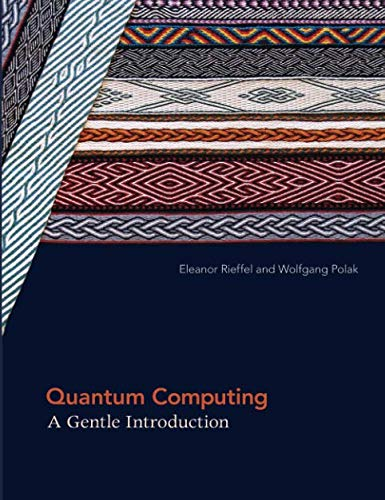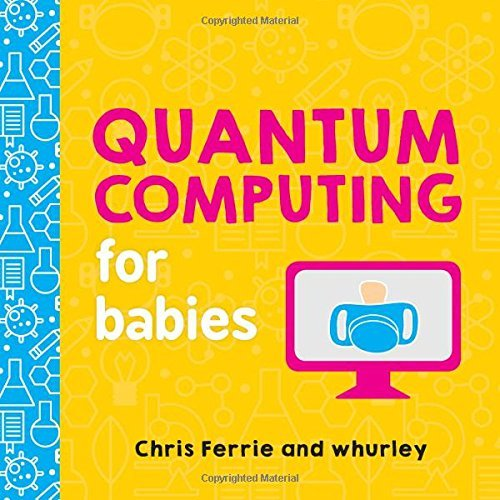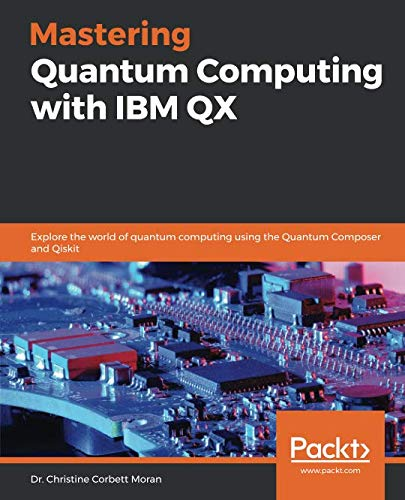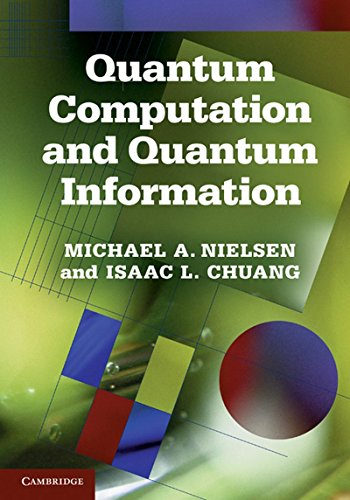# Best Quantum Computing Books 2020 | Learn Quantum Computing

Quantum computing applies the properties of quantum mechanics such as superposition and entanglement to process information. The computation must be implemented theoretically or physically.

In the following, you will get some Best quantum computing books 2020

## 1. Quantum Computing for Everyone (The MIT Press)

If you want to jump into the world of quantum computing then Quantum Computing for Everyone is for you. This book is very clear to understand and is an entry-level book. Prior knowledge of linear algebra is required if anyone wants to understand the basics of how and why a quantum computer works and some of the concepts of Quantum Mechanics.

In this book, Chris Bernhardt explained

• An introduction to quantum computing
• The basic unit of quantum computing
• How the qubit can be measured
• Qubits, entanglement, quantum teleportation
• Quantum gates
• The speed of quantum algorithms
• The building of quantum computers
• Quantum algorithms.

## 2. Quantum Computing: A Gentle Introduction (Scientific and Engineering Computation)Author: Eleanor G. Rieffel,Wolfgang H. Polak
Published at: 29/08/2014
ISBN: 0262526670

Quantum Computing: A Gentle Introduction is an excellent book containing exercises which are useful, explanations are well thought out and written. Full of information, examples, and formulas. This quantum computing books also contains the necessary information to understand programming.

This book provides you the combination of two of the twentieth century's most influential topics like

• Revolutionary scientific theories
• Information theory and
• Quantum mechanics

It offers you a thorough exposition of quantum computing and the underlying concepts of quantum physics, explaining all the relevant mathematics and offering numerous examples. Students and professionals in mathematics, computer science, and engineering can access to the quantum computing of this book.

## 3. Quantum Computing since Democritus

People having some knowledge in math like complex numbers, probability, and matrices (linear algebra), physics and computer science will find Quantum Computing since Democritus very beneficial. Those who are interested in philosophy, life, and the universe will definitely enjoy the best quantum computing books.

This book offers you

• Logic and set theory
• Computability and complexity theory
• Quantum computing
• Cryptography
• The information content of quantum states
• The interpretation of quantum mechanics
• Time travel,
• The anthropic principle
• The views of Roger Penrose.

## 4. Quantum Computing for Babies (Baby University)Author: Chris Ferrie,whurley
Published at: 03/04/2018
ISBN: 1492671185

Quantum Computing for Babies provides a simple and straightforward explanation. It offers your younger daughter or sister a great way to learn quantum computing. All the complex ideas are explained simply.

You'll discover

• The difference between bits and qubits
• How quantum computers will change our future
• Bigger vocabulary and exposure to complex concepts.

## 5. Mastering Quantum Computing with IBM QX: Explore the world of quantum computing using the Quantum Composer and QiskitAuthor: Dr. Christine Corbett Moran
Published at: 31/01/2019
ISBN: 1789136431

This book provides you the core concepts and principles of quantum computing. You will know the area where quantum principles can be applied. Again you'll be able to design programs with quantum logic. This book shows you the way of developing the ability to analyze the potential of quantum computing in your industry.

Key topics are

• What is quantum computing?
• Qubits
• Quantum states, quantum registers, and measurement
• Quantum gates
• Quantum circuits
• The Quantum composer
• Working with OpenQASM 2.0
• QISKit and quantum computer simulation
• Quantum AND (Toffoli) gates and quantum OR gates
• Grover's algorithm
• Quantum Fourier transform
• Shor's algorithm
• Quantum error correction
• The future of quantum computing.

## 6. Quantum Computation and Quantum InformationAuthor: Michael A. Nielsen Isaac L. Chuang
Published at: 01/01/2013
ISBN: 110761919X

Quantum Computation and Quantum Information is a perfect study guide for those interested in modern Quantum physics. This is a solid reference material for a complex subject.

Key contents include

• Introduction and overview
• Introduction to quantum mechanics
• Introduction to computer science
• Quantum circuits
• The quantum Fourier transform and its applications
• Quantum search algorithms
• Quantum computers: physical realization
• Quantum noise and quantum operations
• Distance measures for quantum information
• Quantum error-correction
• Entropy and information
• Quantum information theory.

Thanks for reading this post. If you have any opinion don't hesitate to comment here. Also please subscribe our newsletter to get more updates.